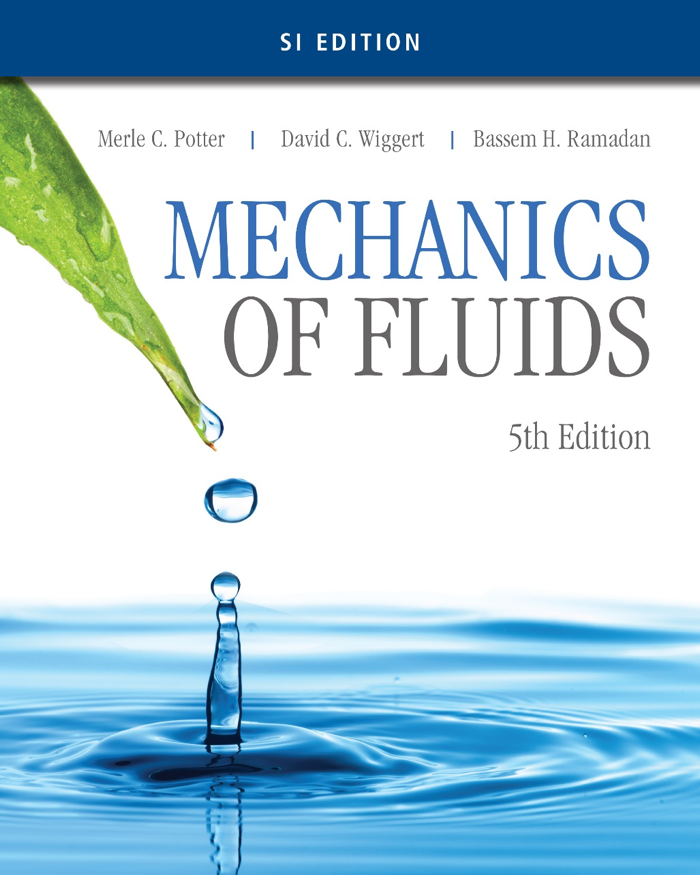• ### COURSE INFORMATION

Open or Close
This course is designed to enhance the basic knowledge that has been developed in the first stage of Fluid Mechanics I (SKMM 2313) and expose the students in analysing hydrodynamically the flow field. It will emphasize on the analysis and the importance of ideal, boundary layer, and compressible flow in a practical engineering applications. The course will also provide the analysis of flow through fluid machines such as pump and turbine. At the end of the course, students should be able to demonstrate and apply the theory to solve problem related to flow of fluids.

COURSE LEARNING OUTCOMES
1. Calculate fluid dynamics problems using differential forms of the fundamental laws. Deduce expression for stream function and velocity potential for elementary flows and various superposition of these elementary flows using the inviscid theory. Determine pressure and velocity of these flows.
2. Derive expression of boundary layer flow using Von Karman momentum integral equation for laminar and turbulent flow. Calculate drag for flow around flat plate.
3. Develop a set of governing equation and calculate flow process for 1-D compressible flow across normal shock through convergence-divergence nozzles.
4. Illustrate basic function of a pump and turbine, its working principle and function of its major components. Calculate the efficiency of centrifugal pump, Pelton wheel and Francis turbine.
5. Design and analyse fluid mechanics components and/or systems based on given specifications.
• ### SYLLABUS

Open or Close
Differential forms of the fundamental laws:
Continuity equation, momentum equation.

Potential flow theory:
Basic flow equations. Definition, stream function and stream lines, circulation and vorticity.
Velocity potential, Cauchy-Riemann equation. Simple solutions, uniform, source, sink, vortex, doublet flows.
Superposition. Uniform + doublet (Flow around cylinder). Uniform + doublet + vortex (Flow around rotating cylinder).

Boundary layer theory:
Flow around immersed bodies: Drag coefficients, lift and drag on airfoils. Von Kármán integral equation.
Approximate solution to the laminar boundary layer, turbulent boundary layer: Power-law form.
Turbulent boundary layer: Empirical form laminar boundary-layer equations.

Compressible flow:
Introduction, speed of sound and the Mach number.
Isentropic nozzle flow, normal shock wave.

Turbomachinery:
Introduction and classification of fluid machines. Radial-flow pumps. Principle of operation and main components. Elementary theory: Velocity triangle and Euler’s turbomachine equation.
Reaction Turbine: Francis turbine. Principle of operation and main components, velocity triangle and efficiency.
Impulse Turbine: Pelton wheel. Principle of operation and main components, velocity triangle and efficiency.

NOTES

Differential forms of the fundamental laws:
Differential forms - Note 2020

Potential flow theory:
Potential flow - Note 2020

Boundary layer theory:
Boundary layer - Note 2020

Compressible flow:
Compressible flow - Note 2020

Turbomachinery:
Turbomachinery - Note 2020

• ### ASSESSMENT

Open or Close
Test 1 - 20%
Test 2 - 20%

Assignment - 20%

Final Exam - 40%

(Marks percentage may be changed due to Fluid Panel's decision)
• ### REFERENCES

Open or CloseOfficial Text book
Potter, Wiggert, Ramadan. 2016. Mechanics of Fluids, SI Edition, 5th Ed. Cengage Learning.
Other References
1. Munson, Young, Okiishi, Huebsch. 2010. Fundamental of Fluid Mechanics, 6th Ed. John Wiley and Sons.
2. Fox, McDonald and Pritchard, 2004, Introduction to Fluid Mechanics, 6th Edition, John Wiley and Sons.
3. Douglas, Gasiorek, Swaffield, Fluid Mechanics, 4th Edition, Prentice Hall.
4. White. 2016. Fluid Mechanics. 8th Edition. McGraw-Hill International Edition.
5. Massey, Mechanics of Fluids, Chapman & Hall, London.